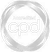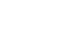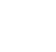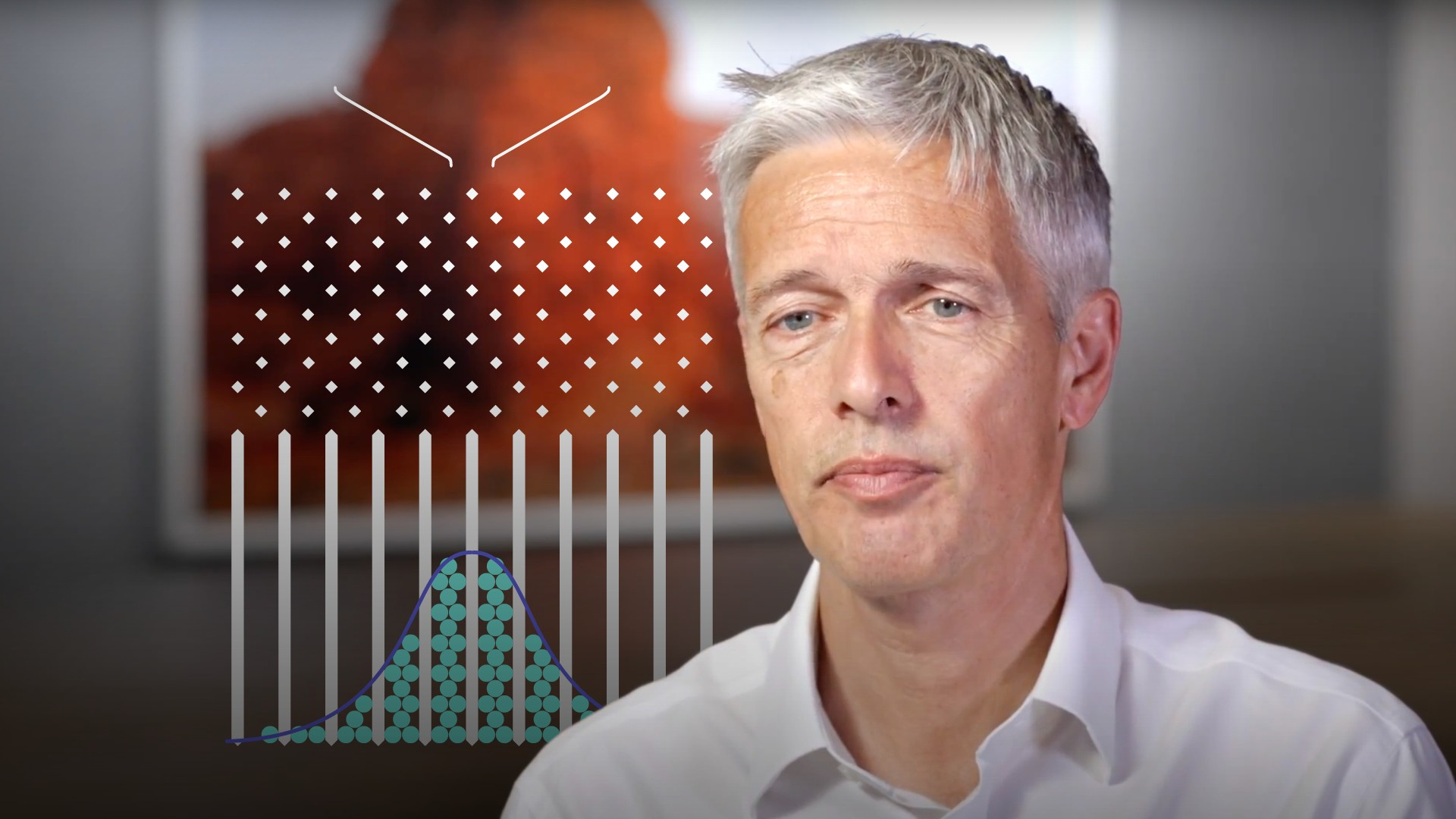# FX Options Pricing35 years: FX & financial markets

In this second part of the introduction to foreign exchange options, Kees discusses how to determine the fair value of an option by assessing the probabilities of option payouts.

Derivatives

Financial Markets

Corporate DerivativesIn this second part of the introduction to foreign exchange options, Kees discusses how to determine the fair value of an option by assessing the probabilities of option payouts.

Finance Unlocked is the video learning platform built for finance professionals.### FX Options Pricing

13 mins 4 secs

Overview

The expected pay-out is equal to the upfront premium and is calculated by multiplying the sum of each of the event payoffs and its probability. In the context of the Fx market, the EP depends on where the underlying price is compared to the strike of this option, these prices are normally distributed. However, payoffs are continuous meaning they are calculated using average payoffs.

Key learning objectives:

• Define expected pay-out and how its calculated in the p.d.f context

• Explain the focus of models, and how expiry prices are distributed to calculate payoffs.

• Identify the reality of calculating EPs in c.r.v form

• Understand the assumptions behind ‘Black Scholes’

## Book a demo to access

Summary

#### What is an Expected Pay out?

EXPECTED PAYOFF = ∑ PROB(EVENT) x PAYOFF(EVENT)

When calculating the payoff for an option, the price of the underlying at expiry must be considered. As mentioned above, the expected payoff is contingent on where the strike price is compared to the market price.

In the case of a call option, we would look at the probability that the spot price is above the strike. All other outcomes are ignored as the option will be left to expire (out of the money). There will be a payoff when the option is in the money.

#### What is the focus of models?

• Where can the spot price move to during the life of an option?
• What is the likelihood of each price event?
• What is the distribution?

#### How are the prices at expiry distributed?

Current spot levels are assumed to either move either up or down by 1 pip. Hence, the next level is the current spot (+ or -) 1 pip.

A normal distribution curve is used as the outcomes form a bell shape. Also, returns will be normally distributed and not on the price of the underlying itself.

If the returns follow a symmetric normal distribution, the distribution of prices follow a lognormal distribution. With this, equal steps in percentage of the price are taken, as opposed to equal steps in size.

#### Given prices at expiry are continuous, how does the Expected Payout calculation differ?

A continuous distribution, not discrete must be considered when looking at spot prices at the expiry as there can be a whole range of values leading to some pay-off. Similarly, an infinite number of outcomes. Instead of looking at every possible outcome, an average payoff is measured.

To calculate the fair value at expiry, the probability to end in the money is multiplied by the average payoff. The chance of ending up in the money is the area under the lognormal distribution curve above the call strike, or, below for a put option.

#### What are Call/Put Options?

• Call option - The average payoff is the average outcome (price at expiry) minus the strike price.
• Put Option – The strike price minus the average payoff.

#### What is the Black Scholes model and what are the assumptions behind it?

The Black Scholes model uses mathematical techniques to put a price on an options contract.

Assumptions:

• Financial prices follow a lognormal distribution
• No transaction costs
• The underlying asset can be bought and sold freely in any quantity
• There are no dividends and no margin requirements
• There is a fixed interest rate
• Standard deviation is considered a constant both over time and strikes.

However, real life is more dynamic and variables that go into the formula to get an option premium like volatility and interest rates are not constant. Also, there are transaction costs (a bid-offer spread) along with occasional jumps in the market.

## Book a demo to access

Expert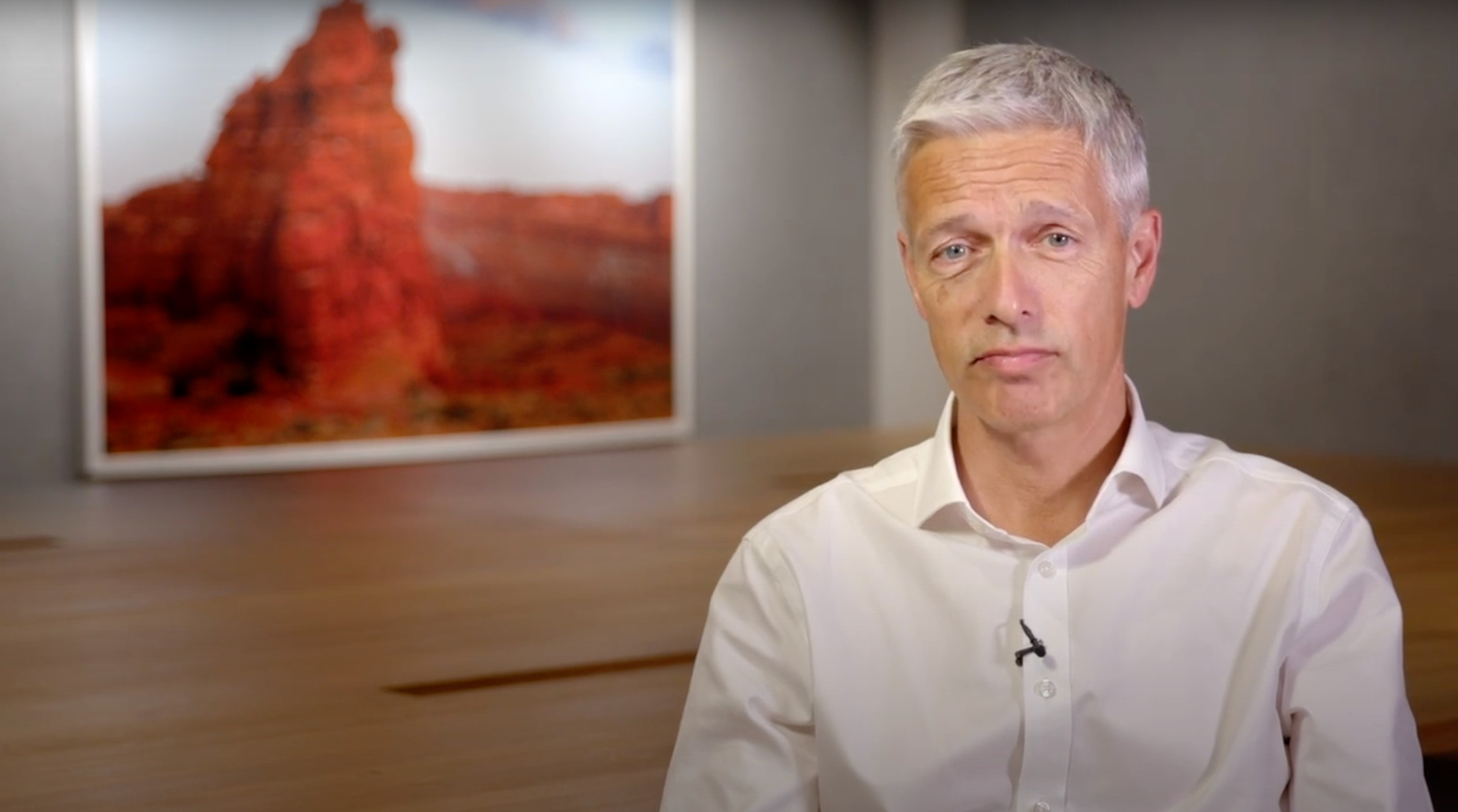Kees has over 30 years of experience in Financial Markets. He has sophisticated expertise in sales and trading roles working in Foreign Exchange and Money Markets. He now conducts seminars, workshops and training courses for clients all over the world.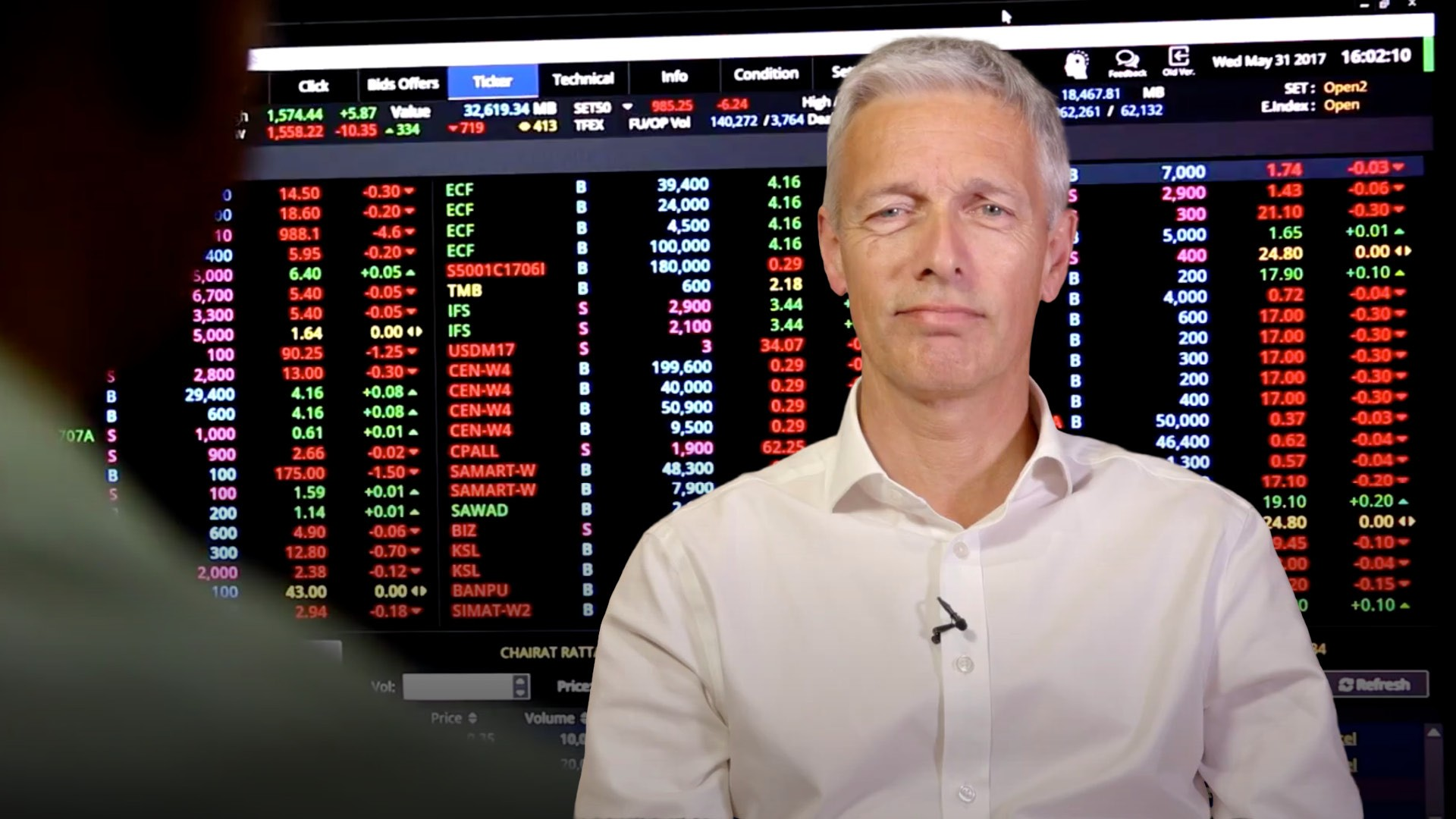17:36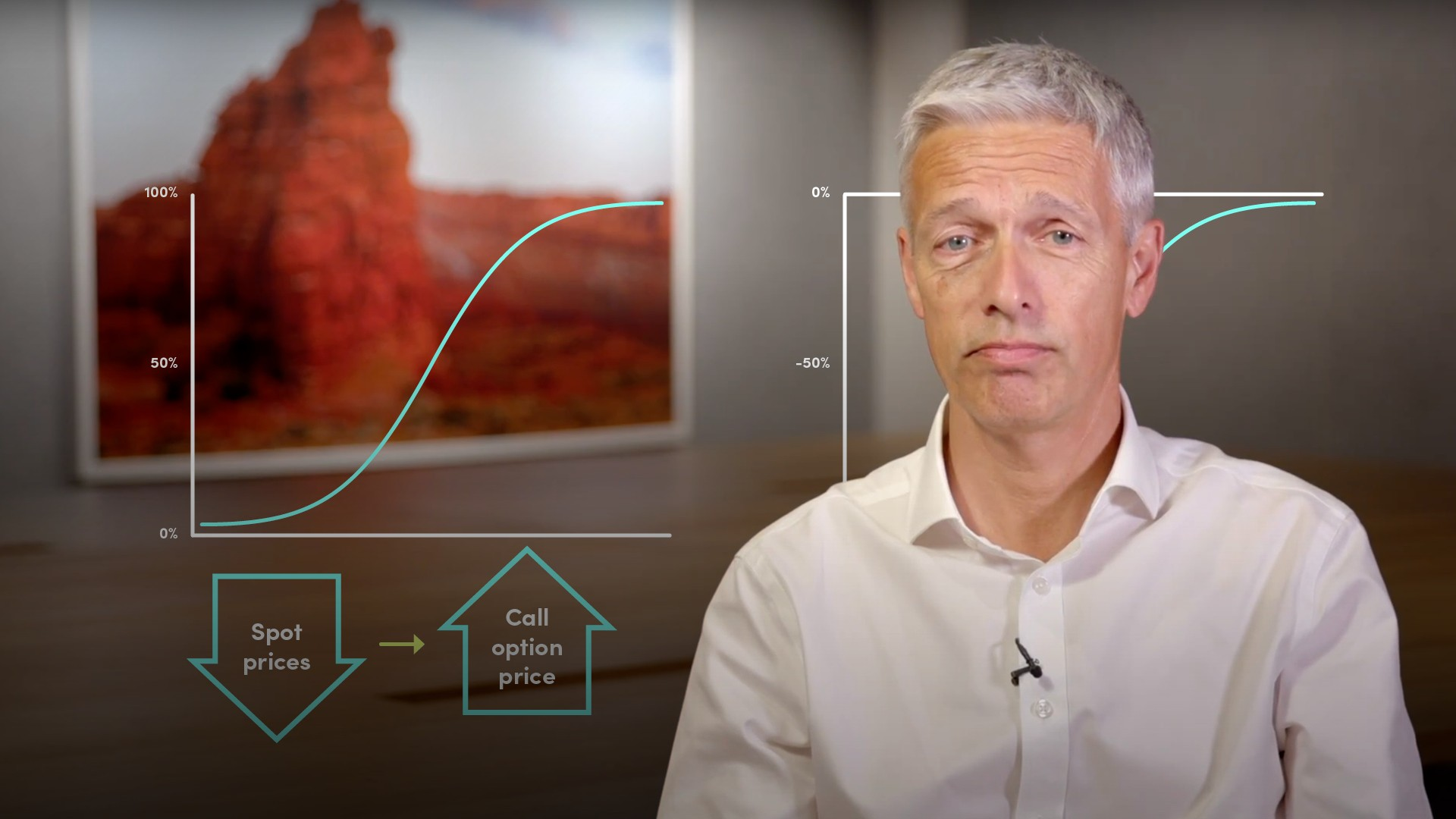16:12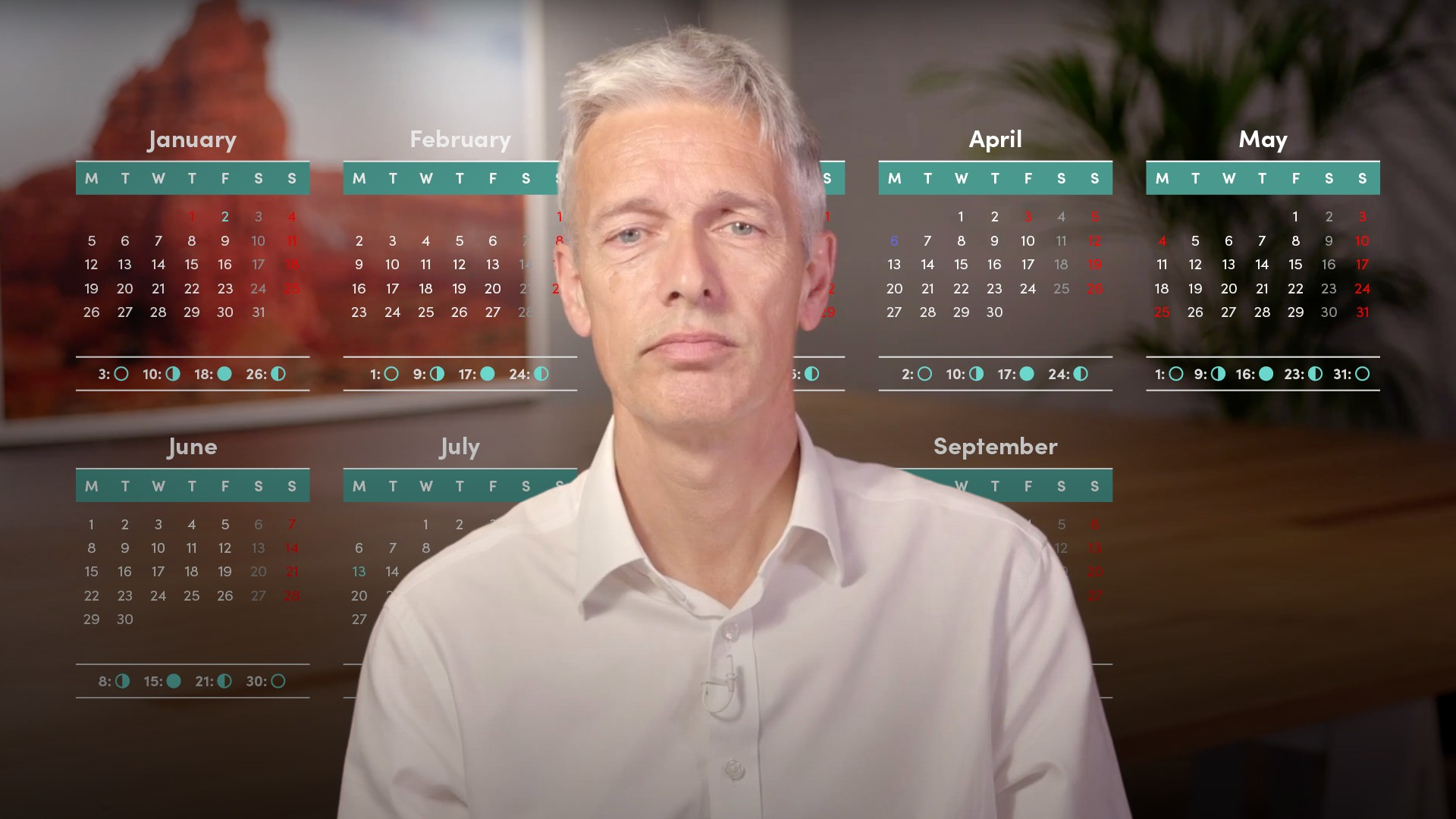12:05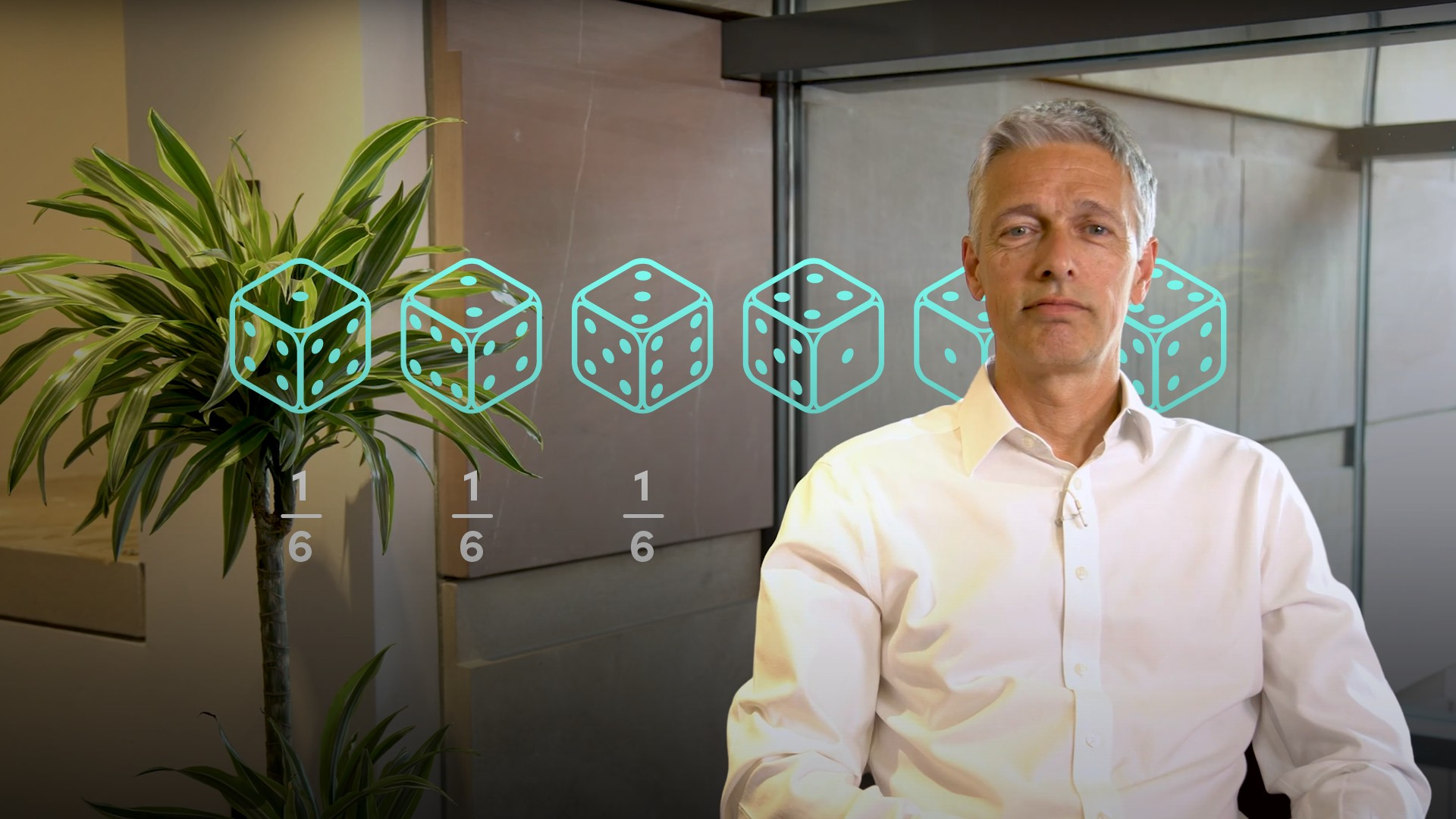21:12

## Related videos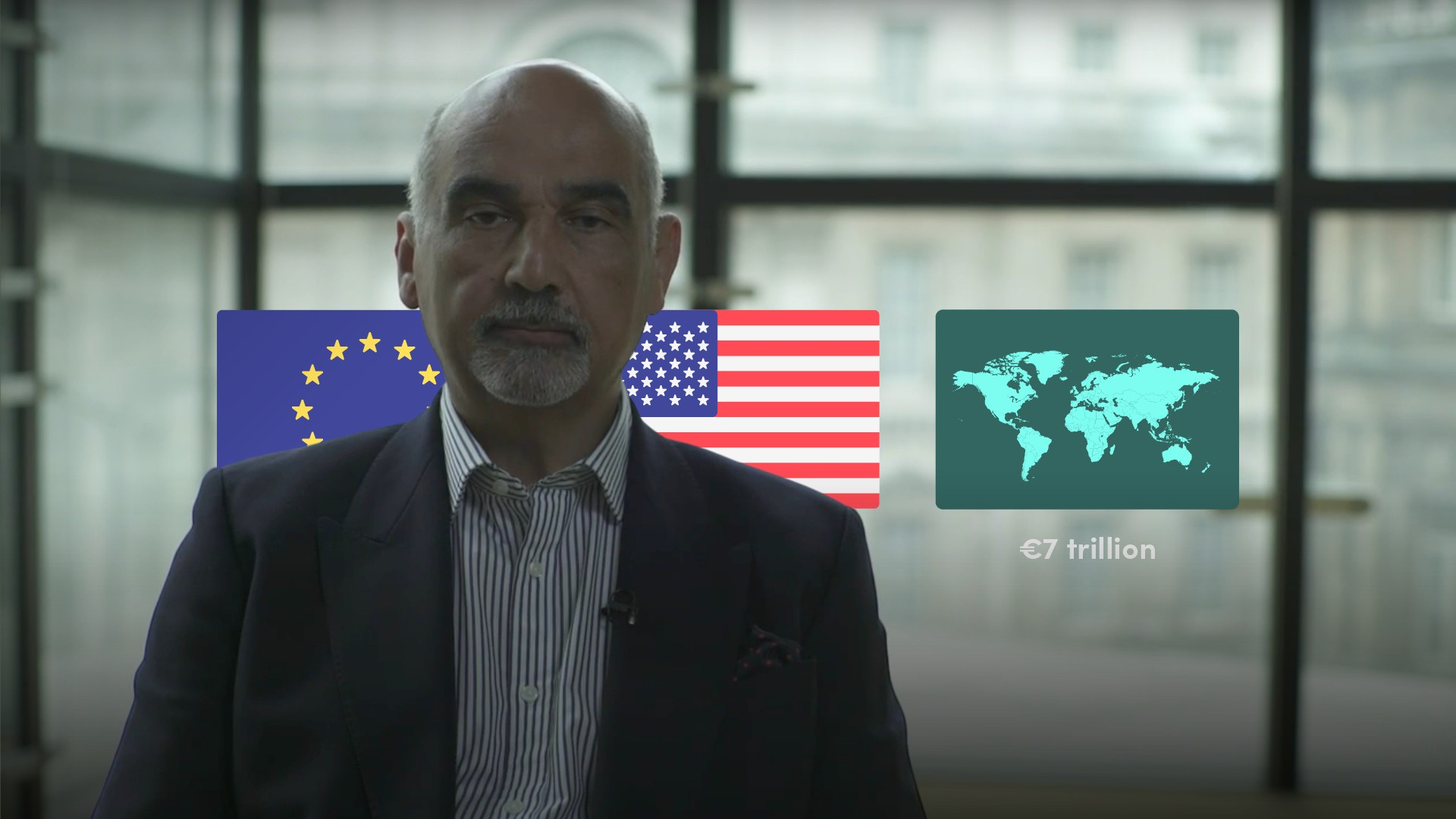Treasury Operations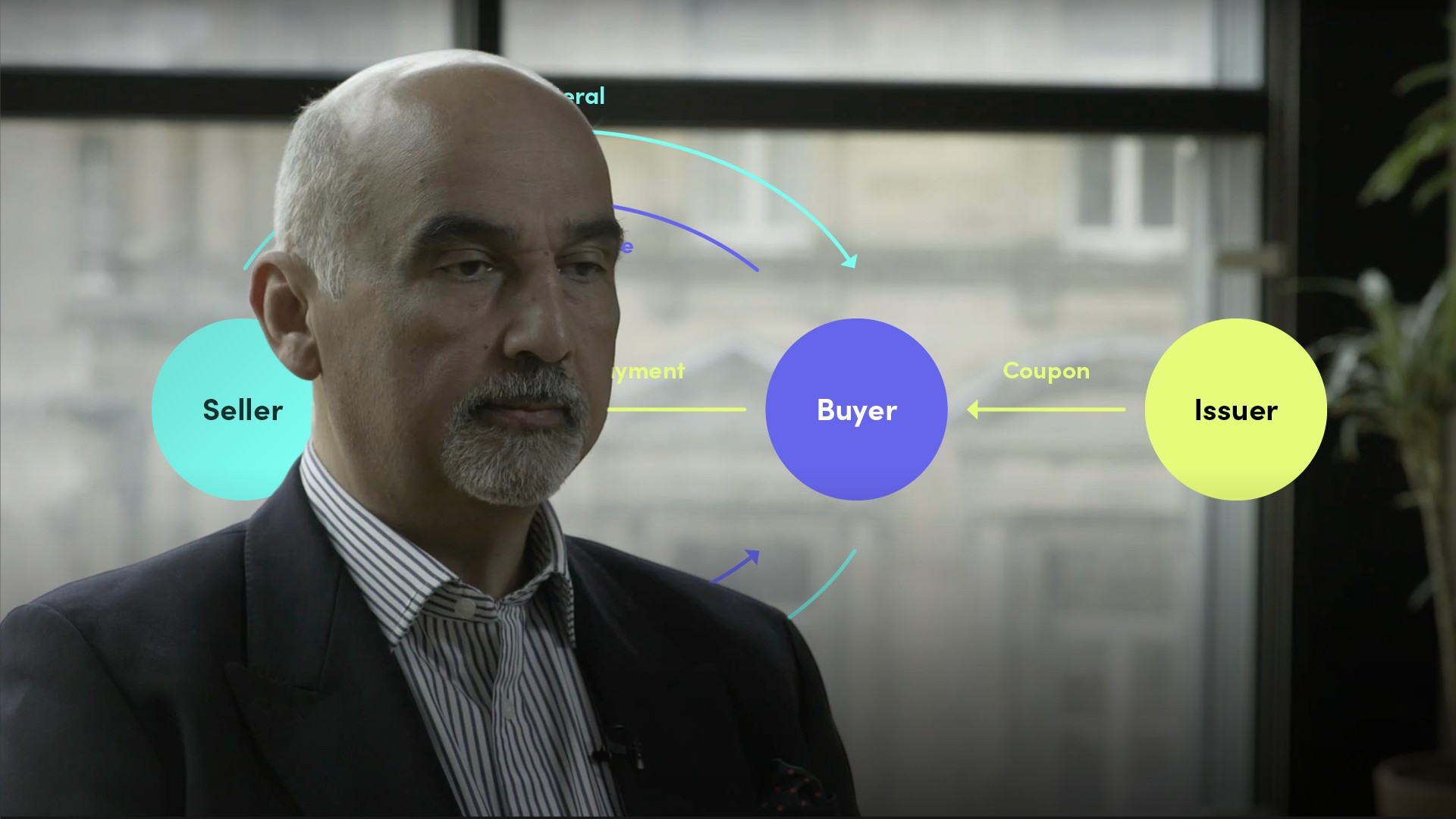Treasury Operations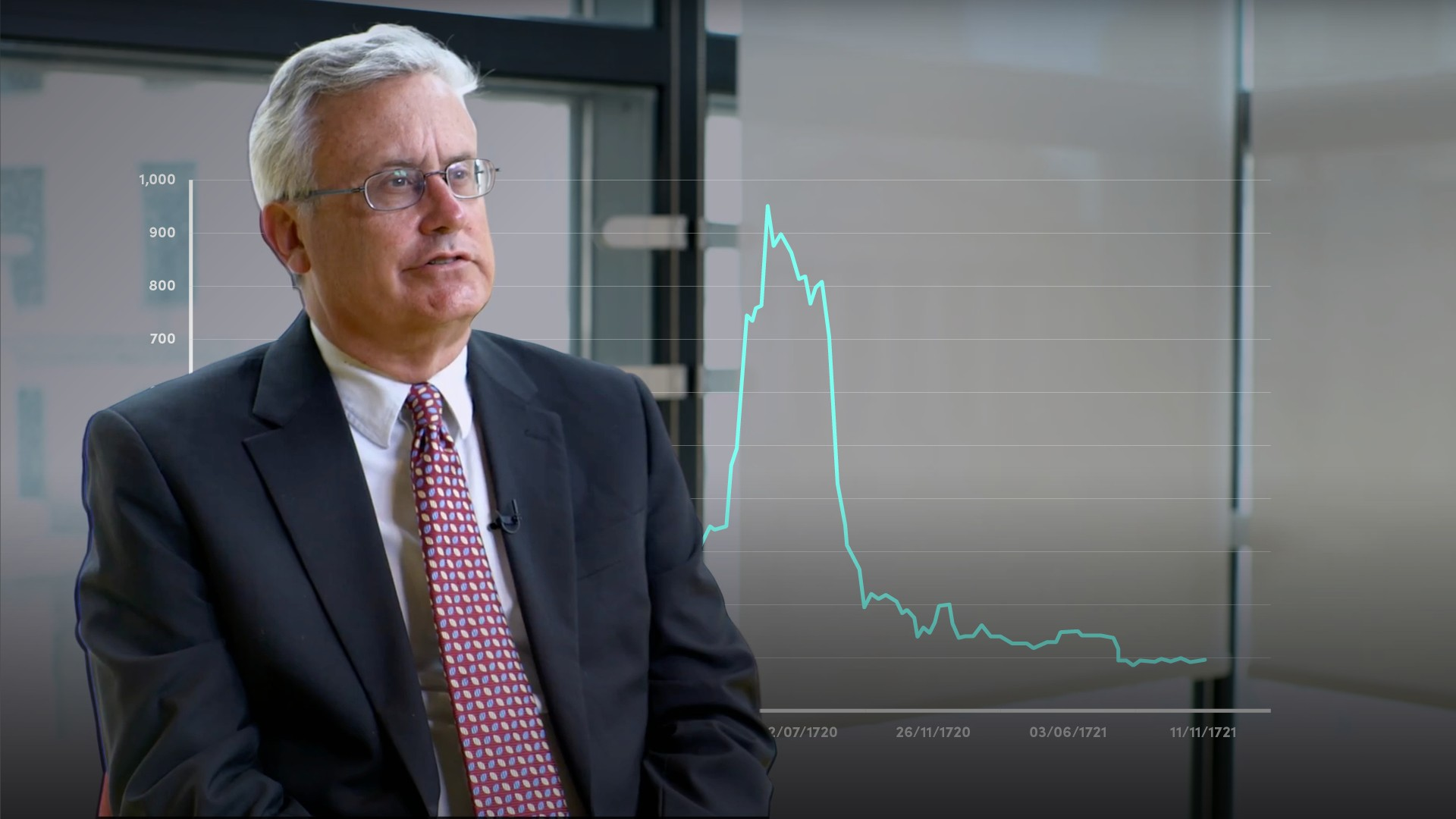Financial Markets### Accredited by